## Abstract

The aim of this contribution was to present a study based on the determination and the visualization of the structural deformations due to the contact of slipping between the disc and the pads. The results of the calculations of the contact described in this work relate to displacements, Von Mises stress on the disc, and contact pressures on the inner and outer pad at various moments of simulation. We first proceed to view the meshed models and predicting variations of tensile or compressive stress normal to the plane and shear stress in rotating disc and ring bodies. One precedes then the influence of some parameters on the computation results such as rotation of the disc, the smoothness of the mesh, the material of the brake pads and the friction coefficient entering the disc and the pads, the number of revolutions and the material of the disc, the pad groove.

## Keywords

Finite element method (FEM); Ventilated disc brake; Gray cast iron; Pads; Total distortion; Shear stress

## 1. Introduction

With the development of new technologies in the automotive industry, vehicles have become more and more efficient. Braking systems should follow the same rhythm. The brake, as a major security organ, constantly arouses great interest to engineers. In addition competition in the automotive field is increasingly harsh, putting pressure on efficiency, reliability, comfort, cost and production time of all automotive systems. For an engineer, the goal is to find the best compromise between the requirements of security, technology and economic constraints. To achieve an optimal design, it should implement all available economic technologies to solve the technical problems, thus complementing experimental studies. In the aerospace and automotive industry, many parts are subjected to simultaneous thermal and mechanical loads, constant of fluctuating. The thermo-mechanical stresses cause deformations and may even damage the systems. For example, in friction braking systems, heat is generated in the disc and brake pads, causing high stresses, deformations and vibrations as cited in . Finite element methods are now widely used to solve structural, fluid, and Multiphysics problems numerically. The methods are used extensively because engineers and scientists can mathematically model and numerically solve very complex problem. Today, finite element methods probably are used for the analysis of every major engineering design and probably in every branch of scientific studies. The method is now used primarily through the application of commercial finite element programs. These programs are used on mainframes, workstations and PCs and are employed to solve very complex problem. Recently, several authors (, , , ,  and ) have been successful in applying the finite element method in their research. In order to use the advantages of both finite volume and finite element methods, new numerical method has been introduced, namely Control Volume based Finite Element Method (CVFEM). Multi-physics problems in complex geometries can be simulated via this powerful method  and . Sheikholeslami et al.  used the CVFEM as an application to solve their numerical problem. In work carried out by Kandelousi  and Sheikholeslami et al. , the same technique was used to solve the governing equations considering both Ferrohydrodynamic (FHD) and Magnetohydrodynamic (MHD) effects. Forced convection heat transfer of ferrofluid in the presence of non-uniform magnetic field was studied by Sheikholeslami et al. . CVFEM was also applied in the numerical investigation. This method was applied in a different field of science ,  and .

Reibenschuh et al.  studied the thermo-mechanical analysis of the brake disc, with an elaborate model to determine the effects of thermal and centrifugal loads on the brake disc and its associated system. Subramanian and Oza  studied ventilated brake disc hub assembly subjected to braking torque and bolt pretension. The induced stresses due to the bolt pretension were found to be negligible compared to the braking torque. Shinde and Borkar  carried out another analysis of the brake disc system using ANSYS software to study the performance of two different pad materials – Ceramic and composite Fiber. This research provided useful design tools and improved braking performance of the disc brake system based on the strength and rigidity criteria.

Jungwirth et al.  carried out a thermo-mechanical coupled analysis of design brake discs and calipers. The simulation model was tested on a brake dynamometer to determine the deformations and its fatigue strength. The study was focused on the mechanical interactions between the calipers and brake disc, including the influence of heat power distribution on the brake disc. In work carried out by Söderberg and Andersson  a three-dimensional finite element model of the brake pad and the rotor was developed primarily for the calculations of the contact pressure distribution of the pad onto the rotor. Abdullah et al.  used the finite element method to study the contact pressure and stresses during the full engagement period of clutches using different contact algorithms. In this study, the sensitivity of the results of the contact pressure was exposed to show the importance of the contact stiffness between contact surfaces. Dhiyaneswaran and Amirthagadeswaran  guided a comparative study of disc brake with two different materials. The disc brake model was analyzed in dynamic load conditions and the contact stress pattern was modeled. The displacement and the elastic constraints of the existing material and alternative materials of the disc brake were also compared. Kumar and Vinodh  proposed a new automotive brake rotor design after they compared it with the ventilated disc rotor. The work used finite element analysis for both static structural and thermal transient analyses in order to evaluate and compare their performances. The analysis of the deformations of the rotor under extreme loads was carried out using a static structural analysis method.

Belhocine and Bouchetara  used the finite element software ANSYS 11.0 to study the thermal behavior of full and ventilated disc brake rotor. A transitory analysis of the structural thermo-mechanical couple was employed in order to visualize the stress fields of the constraints and their deformations in the disc. The contact pressure distribution on the brake pad was also established. Belhocine et al.  investigated the structural and contact behaviors of the brake disc and pads during the braking phase in the design case using FE approach, with and without thermal effects. The results of thermo-elastic coupling on Von Mises stress, contact pressures and total deformations of the disc and pads were presented. These are useful in the brake design process for the automobile industry.

In another study by the same authors as, Belhocine et al.  on structural and contact analyses of disc brake Assembly during a single stop braking event using the same commercial software, the stress concentrations, structural deformations and contact pressure of brake disc and pads were examined.

The principal objective of this paper was to develop a three-dimensional (3D) numerical model for the mechanical behavior of an automotive disc brake pad under dry contact slipping conditions during the braking process. The calculations were based on the static structural rested analysis in ANSYS 11.0. The main strategy of the analysis is to initially visualize the normal constraints and shear stresses and thus the sensitivity of some of the computation results, which will then be approached in detail. Thus, this study provides effective reference for design and engineering application of the brake disc and brake pad.

## 2. Study of mechanical contact – brake disc–pad

The disc and the pad were modeled by characterizing the mechanical properties of materials of each part. The type of analysis chosen was the static structural simulation. The total simulation time for braking was t = 45 s, and the following initial time steps were adopted:

• Increment of initial time = 0.25 s.
• Increment of minimal initial time = 0.125 s.
• Increment of maximal initial time = 0.5 s.

### 2.1. ANSYS simulation of the problem

The finite element code ANSYS 11 (3D) was used to simulate the behavior of the contact friction mechanism of the two bodies (pad wafer and disc) during a braking stop. This code has the frictional contact management algorithms based on the Lagrange multipliers method, or the penalization method. The Young’s modulus of the disc was about 138 times higher than that of the pads. The simulations presented in this study, are considered the frictional pad to be deformable pad on a rigid disc (see Fig. 1).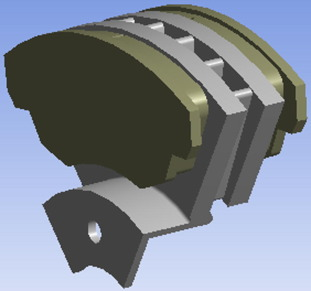Figure 1. Simulation model of a ventilated disc brake and pads.

The application of the contact pressure on the brake pad was input as frictional contact data, and the disc rotational speed was kept constant during the entire simulation. The material chosen for the disc was gray cast iron FG15 high carbon content steel. The brake pad was considered to be made of an isotropic elastic material. The overall mechanical characteristics of the two parts are summarized in Table 1. Parts’ design features are provided by the ANSYS package, whose data are given in Table 2.

Table 1. Mechanical characteristics of the two brake parts.
Young’s modulus, E (GPa) 138 1
Poisson’s ratio, υ 0.3 0.25
Density, ρ (kg/m3) 7250 1400
Coefficient of friction, μ 0.2 0.2

Table 2. Design characteristics of the two brake parts.
Volume (m3) 9.57e−4 8.55e−5
Surface area (m2) 0.242 0.018
Mass (kg) 6.938 0.500
Faces 205 35
Edges 785 96
Summits 504 64
Nodes 34799 2165
Elements 18268 1014
Inertia moment Ip1 (kg m2) 35.776e−3 0.027e−3
Inertia moment Ip2 (kg m2) 69.597e−3 0.151e−3
Inertia moment Ip3 (kg m2) 35.774e−3 0.129e−3

The friction coefficient is 0.2 in the contact zone. Friction is the product of the inter-surface shear stress and the contact area and it is a very complicated phenomenon arising at the contact interface. The coefficient of friction is actually a function of many parameters such as pressure, sliding speed, temperature and humidity. ANSYS is capable of using various methods to solve for the coefficient of friction, such as the Lagrange multipliers, the augmented Lagrangian method, or the penalty method . The latter was selected for this work.

## 3. Modeling of the contact of disc to brake-pad

The following assumptions were made when modeling the brake rotor in the finite element calculations:

• The brake pressure was uniformly distributed over the contact area of the disc and pads.
• The friction coefficient remains constant during braking.
• The materials of the disc and pads are homogeneous and their properties are invariable with temperature.

The loadings and boundary conditions of the finite element model were such that the following conditions were imposed (Fig. 2):

• The disc rotation was assumed constant at ω = 157.89 rad/s according to .
• The cylindrical support is used where axial and radial direction is fixed; and tangential direction free.
• The disc is attached to the wheel hub by 6 bolts through 6 holes, which keep the disc fixed in the three dimensional space.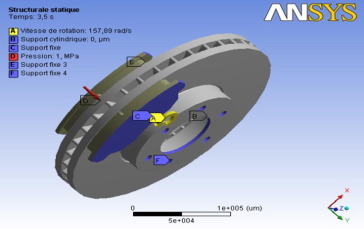Figure 2. Boundary conditions and loading imposed on the disc–pad interface.

The boundary conditions applied to the pads are defined based on the movements allowed by the brake housing mounting that is the side motion. Indeed, the mounting allowed the brake housing and the pads to follow the movement of the disc when the two structures are in contact. The brake housing also holds the pads in the z direction.

The mechanical loading was represented by the plates which were in turn pressed by the brake hydraulic pistons. The pads press the disc, which generated friction on the rotating disc. The clamping force of the plates comes from the cylindrical hydraulic pistons.

Thus, the conditions imposed on the pads were as follows:

• The pad is clamped at its edges in the orthogonal plane on the contact surface, thereby permitting a rigid body movement in the normal direction to the contact surface, such that found in automotive brake assembly .
• Fixed support of the outer pad: The mechanical loading is represented by the pads pressing onto the rotating disc, generating friction. The pad clamping force comes from the cylindrical hydraulic brake piston, pressing the metal back of the pads, which was the only load acting on the pads.
• A pressure P of 1 MPa applied to the inner pad.

For all the calculations that involve friction between surfaces, the coefficient of friction μ, comes into play, whose value depends on many factors, including pressure, sliding speed, temperature, humidity and surface roughness between the two surfaces. Thus the real value varies during braking. For simplicity, it was assumed to be constant and a value of μ = 0.2 was used in all the calculations in this study.

## 4. Mechanical calculation results and discussion

ANSYS computer code also allows the determination and visualization of the structural deformations due to sliding contact between the disc and the pads. The results of the contact calculations described in this section relate to the displacements or the total deformations during the loading sequence, the field of equivalent Von Mises stresses on the disc, and the contact pressures of the outer and inner pads at different moments of the simulation.

### 4.1. Meshing the model

Meshing involves division of the entire model into small pieces called elements. The elements used for the meshed model are tetrahedral 3D elements with 10 nodes. The finite element model of the rotor was carried out such that the resulting elements came to 20351 with a total of 39208 nodes. The meshing of the disc–pad as modeled in ANSYS is presented in Fig. 3.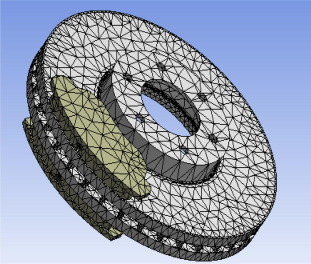Figure 3. Voluminal meshing of the disc and pads giving the total number of nodes of 39208 and number of elements of 20351.

### 4.2. Results of meshed models

A convergence test is intended to evaluate the influence of the mesh on the accuracy of the numerical simulation. Four cases of meshing were tried (coarse, fine, quadrilateral and hexahedral) whose characteristics are shown in Table 3, and Fig. 4.

Table 3. Results of the different cases of meshing.
Mesh type Nodes Elements Element type CPU time (s)
Coarse 39208 20351 SOLID 187 644.234
Quadrilateral 90680 31879 SOLID 186/187 3030.047
Hexahedral 103098 36901 SOLID 186/187 4477.625
Fine 160918 88625 SOLID 187 1982.203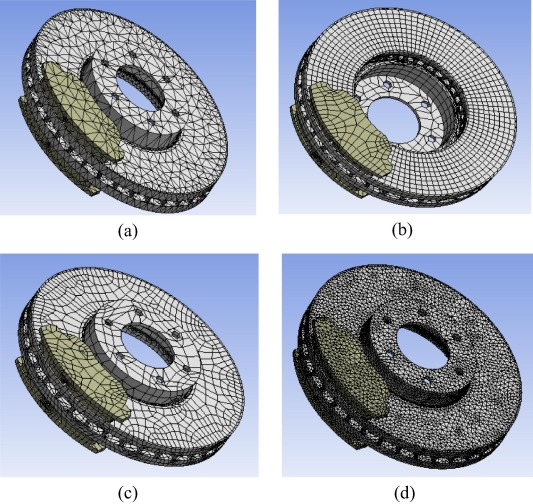Figure 4. Different meshing of the disc, (a) volume mesh (39208 nodes, 20351 elements), (b) quadrilateral elements (90680 nodes, 31879 elements), (c) hexahedral elements (103098 nodes, 36901 elements), and (d) refined mesh (nodes 160918, elements 88625).

Fig. 4 shows the mesh models of the torque of the disc pads.

### 4.3. Tensile/compression and shear stress in the disc

Mechanical properties of materials include the yield strength, tensile strength, fatigue strength, crack resistance, and other characteristics. There are three types of stresses: compressive stress, tensile stress and shear stress. Tensile or compression stresses and shear stresses in the disc are shown in Figure 5 and Figure 6. During the rotation of the disc, there is a concentration of stresses at the fixing holes and the connection area of the tracks to the bowl. Stress is propagated onto the friction track versus time. The maximum value of the compressive stress is in the order of 22.574 MPa and that of tensile stresses of 22.713 MPa. Shear stresses vary from 0.336 MPa to 5.71 MPa. This loading format has an influence on the total deformations of the disc, which could take the shape of a cone. Indeed, the coning mode occurred when the residual stress at the outer radius was tensile and the potato chip mode occurred when it was compressive.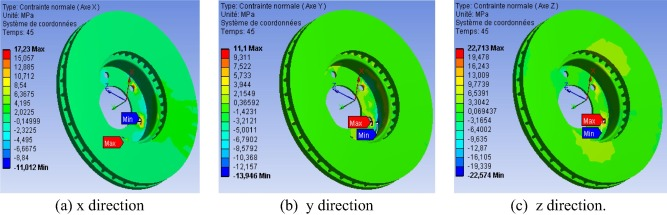Figure 5. Contours of normal stresses in the disc at t = 5 s.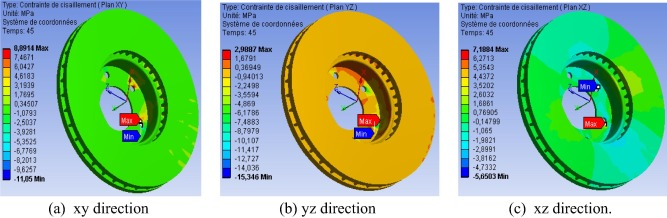Figure 6. Contours of shear stresses in the disc at t = 5 s.

### 4.4. Case of a disc without rotation

Assuming the case of a disc at rest, it is noted that according to Fig. 7, the Von Mises stress concentrations are located only in the bowl, but it does not spread onto the friction tracks, contrary to the case of disc with rotation. The total deformation varies from 0 to 49.58 μm as shown in Fig. 8. There is a difference of 3.24 μm compared to that of the rotating disc. The displacement is located on the outer ring of the disc and reaches the maximum value of 17.68 μm at the periphery of the crown.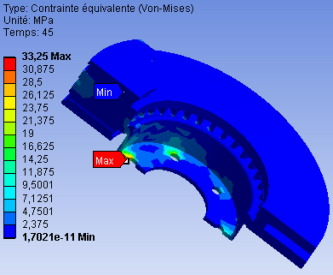Figure 7. Von Mises stress.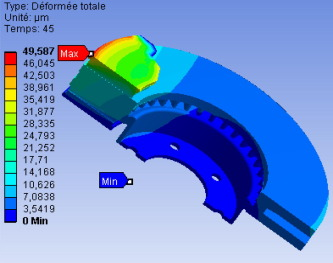Figure 8. Total deformation.

The displacements of the nodes with rotational positions, for points located on the mean outer radius and the outer ring of the disc, with and without rotation are shown in Fig. 9. It is noted that the two curves follow the same pace. A clear difference in disc deformation can be found between these two regions, where the outer radius of the disc shows higher deformation than the mean radius. The curves indicate an umbrella phenomenon that results from the loading of non-parallel paths of friction with respect to the initial position. The maximum displacement value is reached at the angle θ = 90° which corresponds to the position of tightening of the disc by pads. Behavior of displacements with or without rotation is entirely consistent with the observations made with brake discs.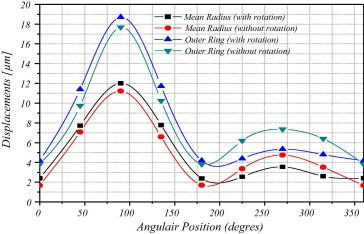Figure 9. Disc displacements at the mean outer radius and outer ring over angle positions.

Fig. 10 shows the reaction forces on the disc, which faced the inner and outer pads in the case with and without disc rotation. The introduction of disc rotation generates an increase in the friction force that is approximately at the contact track. For the external track, it is found that in the case of the rotating disc, the reaction force increases from 2.1 kN to 5.1 kN, and for the interior track from 2.1 kN to 5.9 kN. The differences in the reaction forces (with and without rotation) are very visible, reaching a maximum value of around 4 kN.Figure 10. Variation of the reaction force on the disc with time.

Fig. 11 shows the distribution of contact forces in three dimensions for both cases (with and without rotation). The dominant forces are in the z-direction.Figure 11. Reaction forces on the inner track of the disc.

Tensile or compression stresses and shear stresses are shown in Figure 12 and Figure 13 respectively. The stress results are stressed without rotation, showing a maximum compressive stress of 22.99 MPa in Fig. 11(c). Shear stresses vary from 3.75 MPa to 16.36 MPa.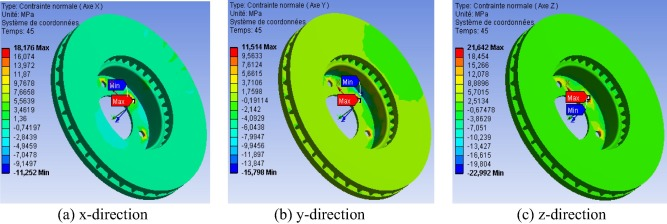Figure 12. Contours of equal normal stresses in the disc at t = 45 s.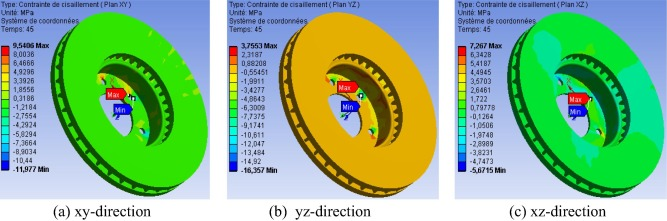Figure 13. Contours of equal shear stresses in the disc at t = 45 s.

Taking account of the effects of the rotation of the disc is essential since it has several effects:

• The maximum stress on the tracks of the disc increases significantly, but they relate to an asymmetrical zone.
• The shear stresses appear at the bowl.

Table 4 summarizes the results of the simulation without disc rotation. In comparison with the rotating disc, there is an increase in stress and decrease in displacements, pressure and friction stresses.

Table 4. Results of the numerical simulation.
Min Max
Total distortion (μm) 0 49.587
σxx (MPa) −11.252 18.176
σyy (MPa) −15.798 11.514
σzz (MPa) −22.992 21.642
σxy (MPa) −11.977 9.540
σyz (MPa) −16.357 3.755
σxz (MPa) −5.671 7.267
Von Mises (MPa) 1.70e−11 33.251
Constraints of friction (MPa) 0 0.281
Sliding distance (μm) 0 3.560
Pressure (MPa) 0 1.755
CPU time (s) 586.656

Figure 14 and Figure 15 show the total deformations and the equivalent Von Mises stresses with and without disc rotation respectively, with respect to time of the simulation. The shape of the curves is similar with increasing differences with time. The deformations of the rotating disc are larger than those without rotation and conversely for the case of von Mises stresses.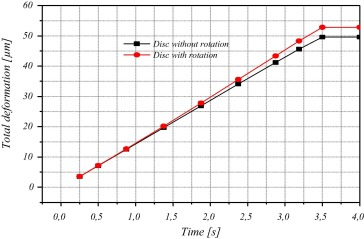Figure 14. Effects of rotation on disc displacement.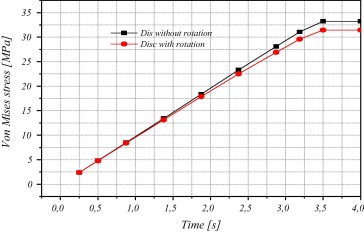Figure 15. Rotator disc on the stress field.

Table 5 shows the maximum Von Mises equivalent stresses and displacement of nodes. The results of the Von Mises stress, increased with the number of elements in the mesh, but the increments were too big, such that the results were questionable. But as the meshing was changed to fine, the results reduce to 44.6 MPa. Therefor the refined mesh should give a more accurate result, was chosen for further analysis of the system.

Table 5. Von Mises stress and total distortion.
Mesh method No. of nodes No. of elements Total distortion (μm) Von Mises stress (MPa) CPU time (s)
Min Max Min Max
Coarse 39208 20351 0 52.829 1.79e−11 31.441 644.234
Quadrilateral 90680 31879 0 55.247 1.99e−02 54.337 3030.047
Hexahedral 103098 36901 0 55.443 1.93e−02 96.434 4477.625
Fine 160918 88625 0 54.817 5.27e−12 44.603 1982.203

### 4.5. Influence of fine mesh

Fig. 16 shows the finer meshing to improve the simulations. The mesh was of the second type, finer elements and more refined elements in the friction tracks. The element used in this mesh is SOLID 187 and the total simulation time is 8.33e6 s. This new mesh (M2 type) consists of 113367 of TE elements with 4 nodes, with 185901 nodes. This is a finer mesh than the M1 mesh used in Fig. 4(d).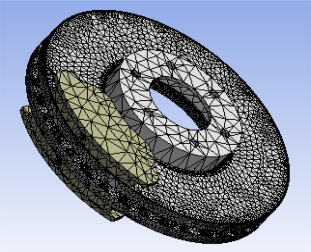Figure 16. Finest mesh with 185901 nodes and 113367 elements.

Table 6 shows the numerical results for the two types of mesh (coarse and fine). It is observed that all the results of extreme values increase with the number of nodes and the number of elements in the mesh. We note the effects of refinement of the mesh impact significantly upon the accuracy of the numerical simulation adopted.

Table 6. Comparison between the results of the fine mesh and finest mesh.
Fine mesh Finest mesh
Nodes Elements Nodes Elements
160918 88625 185901 113367
Min Max Min Max
Total distortion (μm) 0 54.82 0 54.81
Von Mises stress (MPa) 5.27e−12 44.60 18.0e−12 32.48
CPU time (s) 1982.20 8331.33

### 4.6. Influence of pad material

Brake Pad material is a heterogeneous material and is composed of a few elements and each element has its own function. The ideal brake material should have a constant coefficient of friction under various operating conditions such as applied loads, speeds and mode of braking. Here we study the sensitivity of the results compared to two parameters, the Young’s modulus of the brake linings and the friction coefficient between the disc and the pads. The sensitivity study assessed the adequacy of the calculations, but did not take into account the variations of the friction coefficient.

### 4.7. Influence of Young’s modulus of the pad material

Literature review shows that the Young’s modulus of the current pad material generally varies between 0.5 GPa and 1.5 GPa. In this study, we chose two materials whose mechanical and tribological properties are given in Table 7.

Table 7. Mechanical properties of the brake pads.
Material 1 Material 2
Young’s modulus, E (GPa) 1.0 1.5
Poisson ratio, υ 0.25 0.25
Density (kg/m3) 1400 2595
Coefficient of friction, μ 0.2 0.2

Fig. 17 shows the contact state, the contact pressure, frictional stresses and the sliding distance of the inner pad.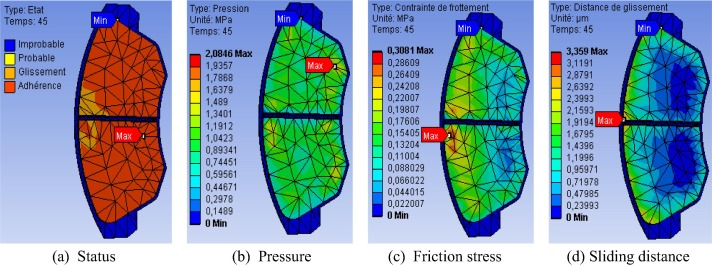Figure 17. Mechanical behavior of the inner pad.

The results of the simulation are summarized in Table 8. The results show that the increase in Young’s modulus of the brake pad causes a reduction in total deformation, stresses (Von Mises, normal and shear), and sliding distance, but increased frictional stresses and contact pressures.

Table 8. Influence of brake pad material (extreme values).
Material 1 Material 2
Min Max Min Max
Total distortion (μm) 0 52.83 0 37.49
σxx (MPa) −11.01 17.23 −8.10 11.34
σyy (MPa) −13.95 11.10 −8.67 7.42
σzz (MPa) −22.57 22.71 −15.51 16.47
σxy (MPa) −11.05 8.89 −7.15 5.83
σyz (MPa) −15.35 2.99 −10.10 1.68
σxz (MPa) −5.65 7.19 −4.38 4.93
Von Mises stress (MPa) 0.00 31.44 0.00 20.88
Friction stress (MPa) 0.00 0.30 0.00 0.31
Sliding distance (μm) 0.00 4.14 0.00 3.36
Pressure (MPa) 0.00 1.79 0.00 2.08
CPU time (s) 644.234 577.000

From Table 8, we can conclude that:

• In the static case, the more flexible pad would result in higher displacements.
• The normal, shear and Von Mises stress, decrease with the increase in the Young’s modulus of the brake pad material.
• On the other hand, the contact pressure and frictional stress increase with the increase in the Young modulus of the brake pads.

Fig. 18 shows the variation of the stresses with change in Young’s modulus of the brake pad material. It is found that the Von Mises stress, normal stresses and shear stresses decrease linearly with the increase in the Young’s modulus of the pad material.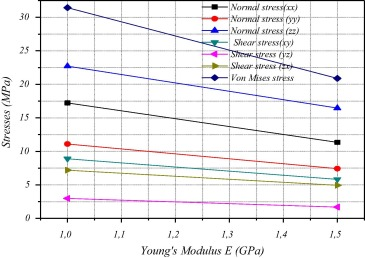Figure 18. Results of stresses with the change in the value of the Young’s modulus of the pad material.

### 4.8. Influence of friction coefficient

Another interest of this study is to understand the sensitivity to variations in the coefficient of friction between the brake pad and the disc. The apparent friction coefficient is the ratio of the tangential force to the normal load applied to a moving tip in contact with the surface of a material. The coefficient of friction value varied from 0.2 to 0.4 in the simulations, to solve for total deformation of the brake model in the final phase of braking. The results are shown in Fig. 19. In the absence of rotation, the results vary very little with changing coefficient of friction. However, with the rotation of the disc, displacements and especially tangential stresses changed substantially.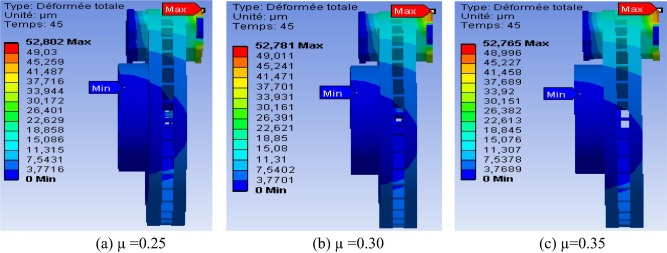Figure 19. Total deformation at the end phase of braking.

Fig. 20 shows the distribution of Von Mises stress field at time t = 3.5 s for two values of friction coefficients. It is found that the friction coefficient does not exert any influence on Von Mises stress in the brake disc.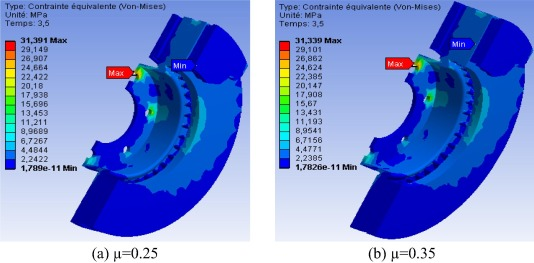Figure 20. Von Mises stresses in the disc at t = 3.5 s.

Fig. 21 shows the resulting contact pressure on the brake pad surface, for different coefficients of friction between the disc and the pads. The results show that the increase in the coefficient of friction is accompanied by a decrease in contact pressures of the pads.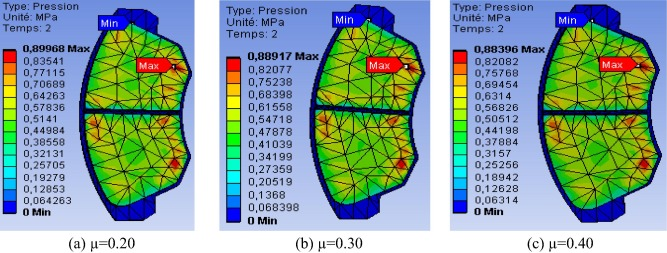Figure 21. Interface contact pressure distribution on the pad at time t = 2 s.

Figure 22 and Figure 23 show the effects of the coefficients of friction on stress and sliding distance in the disc as the time of brake application increased. There is an increase in the friction stress with increased friction coefficient, and the sliding distance is inversely proportional to the coefficient of friction.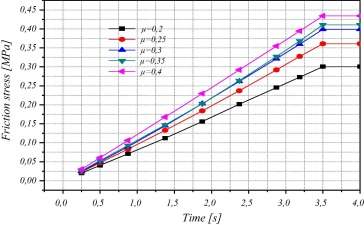Figure 22. Friction stress evolution for various values of μ.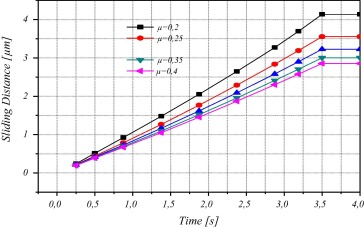Figure 23. Sliding distance evolution for various values of μ.

### 4.9. Influence of the rotational speed of the disc

Fig. 24 shows the contact pressure field at t = 45 s where the maximum pressures were reached at the end of the braking period. It is found that the pressure distribution is almost identical in the three cases and it increases with the increase in the angular velocity of the disc, which agrees with . The location of the maximum pressure is located at the bottom loading edge pad. It is observed that the increase can create higher pad wear, and high pad wear could leave deposits on the disc, resulting in what is called “the third body”. It is noted that the maximum contact pressure in the pad is produced at the leading edge and trailing edge of the friction region.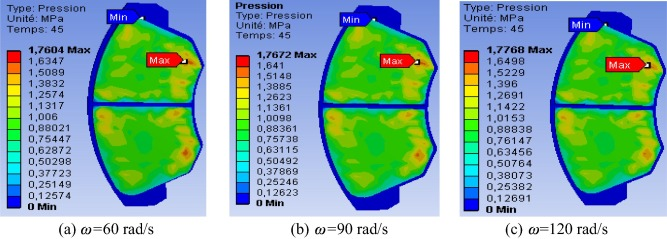Figure 24. Interface contact pressure distributions for different disc speeds.

Fig. 25 shows the distribution of frictional stress at time t = 45 s. It should be noted that the distribution of stress is symmetrical with respect to the pad groove and its value increases slightly when the rotational speed of the disc increases.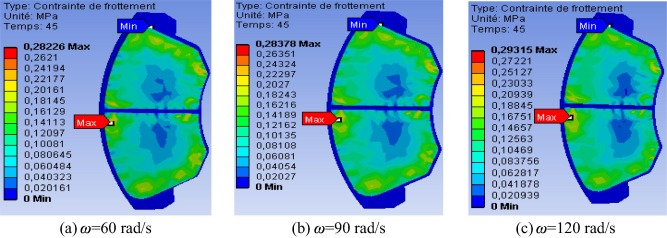Figure 25. Interface friction stress distributions for different disc speeds.

Fig. 26 shows the distribution of equivalent Von Mises stress at the end of braking for various angular velocities of the disc. It is observed that the stress distribution density increases at the inner pads with increasing disc speed. The evolution of Von Mises stresses in the disc surface for different disc speeds is presented in Fig. 27. It is noted that the stress of the disc remains substantially identical and it is inversely proportional to the rotational speed.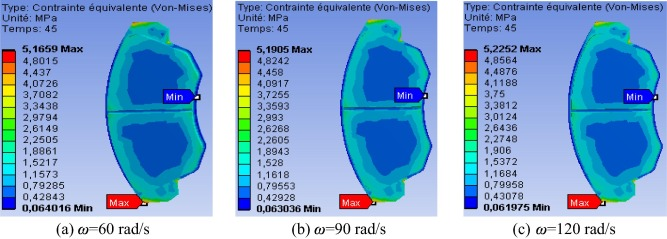Figure 26. Interface friction of Von Mises stress.Figure 27. Influence of the rotational speed on the distribution of Von Mises stress field.

### 4.10. Case of a stainless steel disc

For comparative purposes, another material for the brake disc was studied in place of the original material, while keeping the same material for the pads. The mechanical characteristics of the two parts in contact are summarized in Table 9.

Table 9. Mechanical characteristics of the stainless steel disc, and the brake pads.
Young’s modulus, E (GPa) 203 1
Poisson ratio, υ 0.30 0.25
Density, ρ (kg/m3) 7900 1400
Coefficient of friction, μ 0.2 0.2

### 4.11. Comparison of displacement field

Fig. 28 shows the total deformation of both the disc and the friction pads. It can be seen that the results of the movements of the stainless steel disc coincide exactly with those of gray cast iron. It is observed that the maximum value reached, slightly decreased from 52.83 μm to 51.41 μm (a difference too small, it was assumed negligible).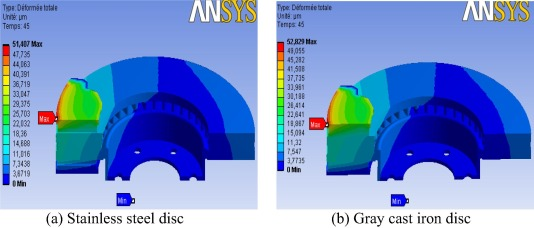Figure 28. Total deformation at the end of braking.

### 4.12. Comparison of stress field

Fig. 29 shows the Von Mises stresses on the stainless steel disc. It can be seen that the distribution of the stresses is very different for different material. In the stainless steel disc, the maximum stress is 43.05 MPa, while in the gray cast iron disc, it is 31.44 MPa. The 11.51 MPa difference is quite large, considering the maximum stress value. The larger maximum stress value in the stainless steel disc (Fig. 29a) means that the stainless steel disc is less efficient in braking function, compared to the gray iron disc (Fig. 29b). This is why gray iron is most commonly used in the automotive industry. The gray iron disc also provides good thermal and mechanical behaviors  (good mechanical strength and low wear).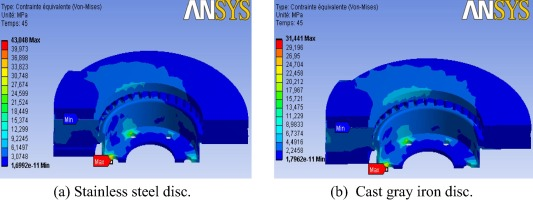Figure 29. Von Mises stress distribution of different disc materials.

The results of the simulations showed that the stainless steel disc had reduced the values of total distortion but suffered higher Von Mises stresses. These are shown in Figure 30 and Figure 31.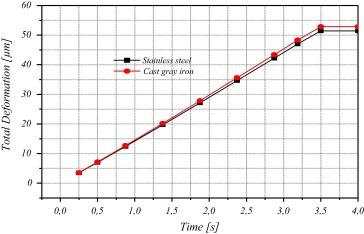Figure 30. Von Mises stress distribution of different disc materials.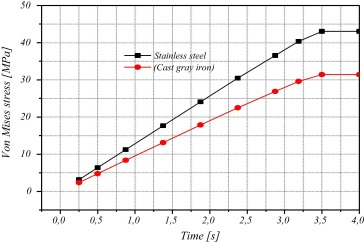Figure 31. Change in the Von Mises stress versus time for two discs.

### 4.13. Study of the influence of the groove

Automotive brake pads usually have median grooves. In addition to removal of dust and water, these grooves may have an influence on the mechanical behavior of the braking system. For this, we conducted a comparison of Von Mises stresses and total deformation of the pad with and without groove, as shown in Fig. 32.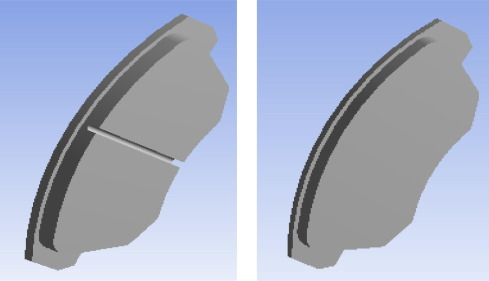Figure 32. Brake pads with and without groove in the pad material.

Fig. 33 shows the Von Mises stresses in the pads and Fig. 34 shows total displacements in the pads, due to the presence of a central groove in the pad material. Von Mises stress and the distortions were lower in the pads without groove. The difference in the Von Mises stress is not really significant, but the difference in distortion is quite significant, i.e. a difference of up to about 10%.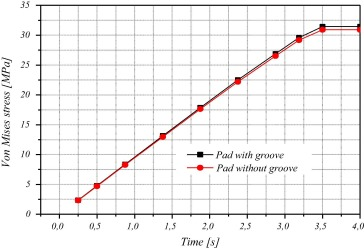Figure 33. Influence of a groove on the variation of the Von Mises stress.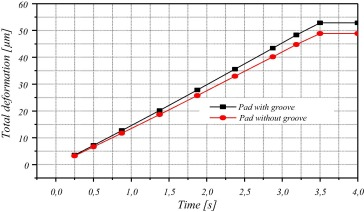Figure 34. Influence of a groove on the variation of the total deformation.

## 5. Conclusions

This work presented a study of purely mechanical dry contact between the brake disc and pads. Using the developed model, the sensitivity of certain parameters could be examined using the FE method and the results could be summarized as follows:

• The parts with high stress concentration are usually found in the disc bowl, the friction door and foot fins, which could cause mechanical failures such as radial cracks, wear, and possibly breakage.
• The rotational speed of the disc has a great influence on the mechanical behavior.
• The most favorable loading is the dual pressure bracket. Finer mesh increases the accuracy of the solution.
• The right choice of pad material depends on its Young’s modulus. The material having the largest modulus of elasticity reduces the maximum stress in the disc, and the disc suffers reduced distortions.
• The choice of pad material would produce a different friction coefficient.
• Increasing the disc rotation speed decreased the equivalent Von Mises stresses, disc shear stress, and results in an increase in the normal constraints of the disc and the friction pressures and stresses and the total deformation of the pads.
• The use of gray cast iron brake discs positively affects the stress on the surface of the disc. It is distinguished by a better mechanical behavior.
• The presence of grooves in the plates negatively affects the mechanical behavior of the brake pad.

### Document informationPublished on 01/01/2017

Licence: Other

### Document Score0

Views 95
Recommendations 0